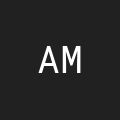# Ask Ben: Looping Through The Days In A Month

By on

How can I loop over the days in a month and do something with each day?

As I have covered before in my blog, looping over dates in ColdFusion is super easy! The beauty of it is that you can treat dates like they are numbers and numbers like they are dates (if it is the right circumstances). All we have to do in this case is start at the first day of the month and loop to the last day in the month:

``````<!---
Create the start day of the month to loop through. We
need to have an actualy month (of a given year) since
of some months (Feb) the number of days per month
changes depending on year.
--->
<cfset dtThisMonth = CreateDate( 2006, 10, 1 ) />

<!---
When looping over the days in a month think about what we
want to do. We want to basically perform an index loop
where on each iteration, the index value is the current
day of the month.

In ColdFusion, dates have a string (ex. Oct 1, 2006) and
a numeric float value (ex. 38991.0) represenation. We can
leverage this to keep adding 1 to the date to get the next
day. And how many days do we add? We keep adding until
we reach the days in the month.
--->
<cfloop
index="dtToday"
from="#dtThisMonth#"
to="#(DateAdd( 'm', 1, dtThisMonth ) - 1)#"
step="1">

<p>
<!--- Display date string. --->
<strong>#DateFormat( dtToday, "mmm d, yyyy" )#</strong><br />

<!---
Display numeric value. Remember, since we are iterating
over the FLOAT values of dates, this should be a float.
--->
Numeric Value: #dtToday#<br />

<!---
Since we have converted the date to its numeric
representation, it is no longer a traditional date,
--->
IsNumericDate(): #IsNumericDate( dtToday )#<br />

<!--- Double check that it is not a traditional date. --->
IsDate(): #IsDate( dtToday )#<br />
</p>

</cfloop>
``````

Notice in the above loop that I am loop to the last day in the current month (subtracting one from the start of the next month). We have to do this because the CFLoop index loop is an INCLUSIVE loop. That means it includes both the start and end indexes of the loop range.

The above example give us:

Oct 1, 2006
Numeric Value: 38991
IsNumericDate(): YES
IsDate(): NO

Oct 2, 2006
Numeric Value: 38992
IsNumericDate(): YES
IsDate(): NO

Oct 3, 2006
Numeric Value: 38993
IsNumericDate(): YES
IsDate(): NO

Oct 4, 2006
Numeric Value: 38994
IsNumericDate(): YES
IsDate(): NO

Oct 5, 2006
Numeric Value: 38995
IsNumericDate(): YES
IsDate(): NO

Oct 6, 2006
Numeric Value: 38996
IsNumericDate(): YES
IsDate(): NO

Oct 7, 2006
Numeric Value: 38997
IsNumericDate(): YES
IsDate(): NO

Oct 8, 2006
Numeric Value: 38998
IsNumericDate(): YES
IsDate(): NO

Oct 9, 2006
Numeric Value: 38999
IsNumericDate(): YES
IsDate(): NO

Oct 10, 2006
Numeric Value: 39000
IsNumericDate(): YES
IsDate(): NO

Oct 11, 2006
Numeric Value: 39001
IsNumericDate(): YES
IsDate(): NO

Oct 12, 2006
Numeric Value: 39002
IsNumericDate(): YES
IsDate(): NO

Oct 13, 2006
Numeric Value: 39003
IsNumericDate(): YES
IsDate(): NO

Oct 14, 2006
Numeric Value: 39004
IsNumericDate(): YES
IsDate(): NO

Oct 15, 2006
Numeric Value: 39005
IsNumericDate(): YES
IsDate(): NO

Oct 16, 2006
Numeric Value: 39006
IsNumericDate(): YES
IsDate(): NO

Oct 17, 2006
Numeric Value: 39007
IsNumericDate(): YES
IsDate(): NO

Oct 18, 2006
Numeric Value: 39008
IsNumericDate(): YES
IsDate(): NO

Oct 19, 2006
Numeric Value: 39009
IsNumericDate(): YES
IsDate(): NO

Oct 20, 2006
Numeric Value: 39010
IsNumericDate(): YES
IsDate(): NO

Oct 21, 2006
Numeric Value: 39011
IsNumericDate(): YES
IsDate(): NO

Oct 22, 2006
Numeric Value: 39012
IsNumericDate(): YES
IsDate(): NO

Oct 23, 2006
Numeric Value: 39013
IsNumericDate(): YES
IsDate(): NO

Oct 24, 2006
Numeric Value: 39014
IsNumericDate(): YES
IsDate(): NO

Oct 25, 2006
Numeric Value: 39015
IsNumericDate(): YES
IsDate(): NO

Oct 26, 2006
Numeric Value: 39016
IsNumericDate(): YES
IsDate(): NO

Oct 27, 2006
Numeric Value: 39017
IsNumericDate(): YES
IsDate(): NO

Oct 28, 2006
Numeric Value: 39018
IsNumericDate(): YES
IsDate(): NO

Oct 29, 2006
Numeric Value: 39019
IsNumericDate(): YES
IsDate(): NO

Oct 30, 2006
Numeric Value: 39020
IsNumericDate(): YES
IsDate(): NO

Oct 31, 2006
Numeric Value: 39021
IsNumericDate(): YES
IsDate(): NO

Want to use code from this post? Check out the license.I'm trying to create a list of days. I'm using this to import historical data. I'm getting some odd results. Duplicate dates mostly. Any insights you can provide would be greatly appreciated :-)

Here is what I have:

<cfloop
index="dtThisyear"
from="2008"
to="#year(now())#"
step="1"
>

<cfloop
index="dtThisMonth"
from="1"
to="12"
step="1"
>

<cfloop
index="dtToday"
from="1"
to="#(DateAdd( 'm', 1, dtThisMonth ) - 1)#"
step="1">

<p>

<cfset thisday = "#dtThisyear#-#dtThisMonth#-#DateFormat( dtToday, "dd" )#" />

<cfif isdate( thisday )>

dtThisDay: <cfoutput>#thisday#</cfoutput><br />

</cfif>

</p>

</cfloop>

</cfloop>

</cfloop>

Oh my chickens, this post is old!